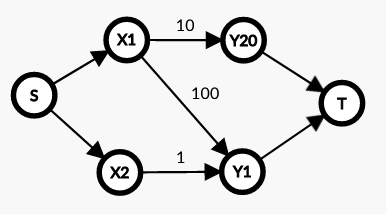Contest Duration: - (local time) (100 minutes) Back to Home

## G - Dream Team Editorial by en_translator

TL; DR: considering a graph where universities and subjects are vertices and people are edges, the original problem boils down to a maximum weight matching problem. A maximum matching problem on a bipartite graph can be solved with minimum cost flow problem.

Construct the following graph.

• Prepare a vertex $$S$$ that represents the source, $$T$$ for the sink, $$X_i$$ for each university, and $$Y_i$$ for each subject.
• Add an edge from $$X_{A_i}$$ to $$Y_{B_i}$$ with capacity $$1$$ and cost $$C_i$$ that represents the $$i$$-th programmer.
• Add edges from $$S$$ to $$X_i$$ and $$X_i$$ to $$T$$ with capacities $$1$$ and costs $$0$$ for each $$i$$.

The graph corresponding to Sample $$1$$ looks like as follows.Here, the maximum sum of power of a dream team consisting of $$i$$ people is the maximum cost of a flow of amount $$i$$ from $$S$$ to $$T$$. By modifying the costs of edges using a sufficiently large constant $$\rm BIG$$, the problem can be transformed into a minimum cost flow problem.

• Add an edge from $$X_{A_i}$$ to $$Y_{B_i}$$ with capacity $$1$$ and cost $${\rm BIG}-C_i$$ that represents the $$i$$-th programmer.

The maximum flow from $$S$$ to $$T$$ is $$M:=\min(\#\{A_i\},\#\{B_i\})$$, so the problem has been solved in a total of $$O(M(M+N)\log(M+N))$$ time.

posted:
last update: